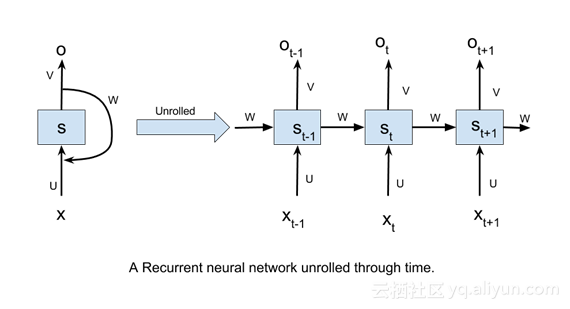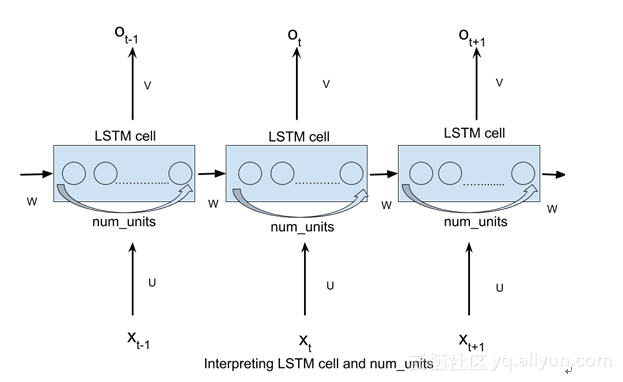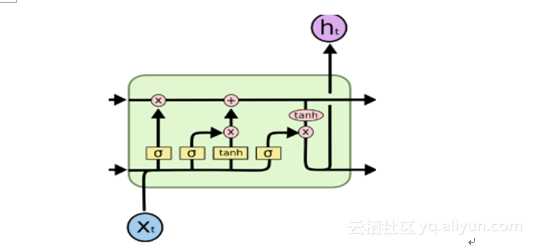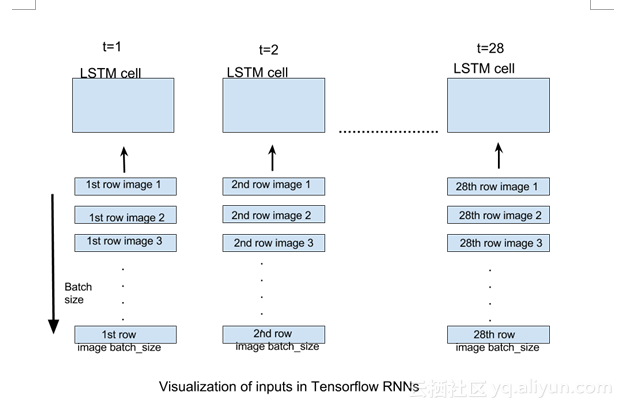# 一步一步带你用TensorFlow玩转LSTM

+关注继续查看

LSTM，全称为长短期记忆网络(Long Short Term Memory networks)，是一种特殊的RNN，能够学习到长期依赖关系。LSTM由Hochreiter & Schmidhuber (1997)提出，许多研究者进行了一系列的工作对其改进并使之发扬光大。

LSTM在解决许多问题上效果非常好，现在被广泛使用。它们主要用于处理序列数据。这个博客的主要目的是让读者了解在TensorFlow中，如何实现基本的LSTM网络并掌握实现的细节。为了实现这一目标，我们把MNIST作为我们的数据集。

MNIST数据集：

MNIST数据集由手写数字及其相应标签的图像组成。我们可以借助TensorFlow的内置功能下载和读取数据：

from tensorflow.examples.tutorials.mnist import input_data


LSTM通常用于解决复杂序列的相关问题，如NLP领域的实验：语言建模、字嵌入，编码器等。MNIST给了我们解决这类问题的机会。这里的输入数据只是一组像素值。我们可以轻松地格式化这些值，并集中应用到问题细节上。

A vanilla RNN1.Xt是指时间步长t的输入。

2.St是指时间步长t处的隐藏状态。它可以被可视化为网络的“内存”。

3.Ot指的是输出在时间步长t。

4.U，V和W是所有时间步长共享的参数。该参数共享的意义在于，我们的模型在不同输入的时间步长可以执行相同的任务。

1.TensorFlow中LSTM细胞的解释。

2.将输入格式化，然后将其输入到TensorFlow RNNs中。

TensorFlow中LSTM细胞的解释：

tf.contrib.rnn.BasicLSTMCell(num_units)

num_units也可以解释为前馈神经网络隐藏层的类比。前馈神经网络隐层中的节点num_units数目等于LSTM网络每个时间步长的LSTM单元的数量。以下图片应该可以帮助你理解：tf.static_rnn(cell,inputs)

当然还有其他形式的定义方法，这里我们只需要用到最简单的定义方法。

inputs参数是为了接受形状张量列表[batch_size,input_size]。该列表的长度是网络展开的时间步长数，即该列表的每个元素对应于我们展开网络的相应时间步长的输入。Code

import tensorflow as tf
from tensorflow.contrib import rnn
#import mnist dataset
from tensorflow.examples.tutorials.mnist import input_data
#define constants
#unrolled through 28 time steps
time_steps=28
#hidden LSTM units
num_units=128
#rows of 28 pixels
n_input=28
learning_rate=0.001
#mnist is meant to be classified in 10 classes(0-9).
n_classes=10
#size of batch
batch_size=128


#weights and biases of appropriate shape to accomplish above task
out_weights=tf.Variable(tf.random_normal([num_units,n_classes]))
out_bias=tf.Variable(tf.random_normal([n_classes]))
#defining placeholders
#input image placeholder
x=tf.placeholder("float",[None,time_steps,n_input])
#input label placeholder
y=tf.placeholder("float",[None,n_classes])


#processing the input tensor from [batch_size,n_steps,n_input] to "time_steps" number of [batch_size,n_input] tensors
input=tf.unstack(x ,time_steps,1)


#defining the network
lstm_layer=rnn.BasicLSTMCell(n_hidden,forget_bias=1)
outputs,_=rnn.static_rnn(lstm_layer,input,dtype="float32")


#converting last output of dimension [batch_size,num_units] to [batch_size,n_classes] by out_weight multiplication
prediction=tf.matmul(outputs[-1],out_weights)+out_bias


#loss_function
loss=tf.reduce_mean(tf.nn.softmax_cross_entropy_with_logits(logits=prediction,labels=y))
#optimization
#model evaluation
correct_prediction=tf.equal(tf.argmax(prediction,1),tf.argmax(y,1))
accuracy=tf.reduce_mean(tf.cast(correct_prediction,tf.float32))


#initialize variables
init=tf.global_variables_initializer()
with tf.Session() as sess:
sess.run(init)
iter=1
while iter<800:
batch_x,batch_y=mnist.train.next_batch(batch_size=batch_size)
batch_x=batch_x.reshape((batch_size,time_steps,n_input))
sess.run(opt, feed_dict={x: batch_x, y: batch_y})
if iter %10==0:
acc=sess.run(accuracy,feed_dict={x:batch_x,y:batch_y})
los=sess.run(loss,feed_dict={x:batch_x,y:batch_y})
print("For iter ",iter)
print("Accuracy ",acc)
print("Loss ",los)
print("__________________")
iter=iter+1


#calculating test accuracy
test_data = mnist.test.images[:128].reshape((-1, time_steps, n_input))
test_label = mnist.test.labels[:128]
print("Testing Accuracy:", sess.run(accuracy, feed_dict={x: test_data, y: test_label}))1126 04398 0Xshell使用SSH远程登录阿里云ECS服务器CentOS7
10121 018619 07735 09407 010760 02024 016738 046769 0
【方向】

696

5

《SaaS模式云原生数据仓库应用场景实践》

《看见新力量：二》电子书